Math resources Algebra Algebraic Expressions

Simplifying Expressions

# Simplifying expressions

Here you will learn about simplifying expressions, including using the distributive property and combining like terms.

Students will first learn about simplifying expressions as part of expressions and equations in 6th grade.

## What is simplifying expressions?

Simplifying expressions involves using the properties of operations to create equivalent algebraic expressions.

For example,

Simplify 4(x+7)-x.

First, look at 4(x+7) which is ‘4 times the sum of x and 7.’

Notice that each method shows 4(x+7)=4x+28.

Since 4(x+7)-x=4x+28-x, you can continue to simplify by combining the like terms, in this case the x s.

Use the commutative property to change the order:

4x+28-x=4x-x+28

Next, let’s simplify the 4x-x .

Visually, if you have 4 positive x ‘s and we subtract an x, you are left with 3 positive x ‘s:

So, 4x-x=3x.

This means that 4x+28-x can be simplified to 3x+28.

You cannot simplify any further because ‘3x’ and ‘+28’ are not like terms. One has the variable x, and the other is a constant.

### What is simplifying expressions?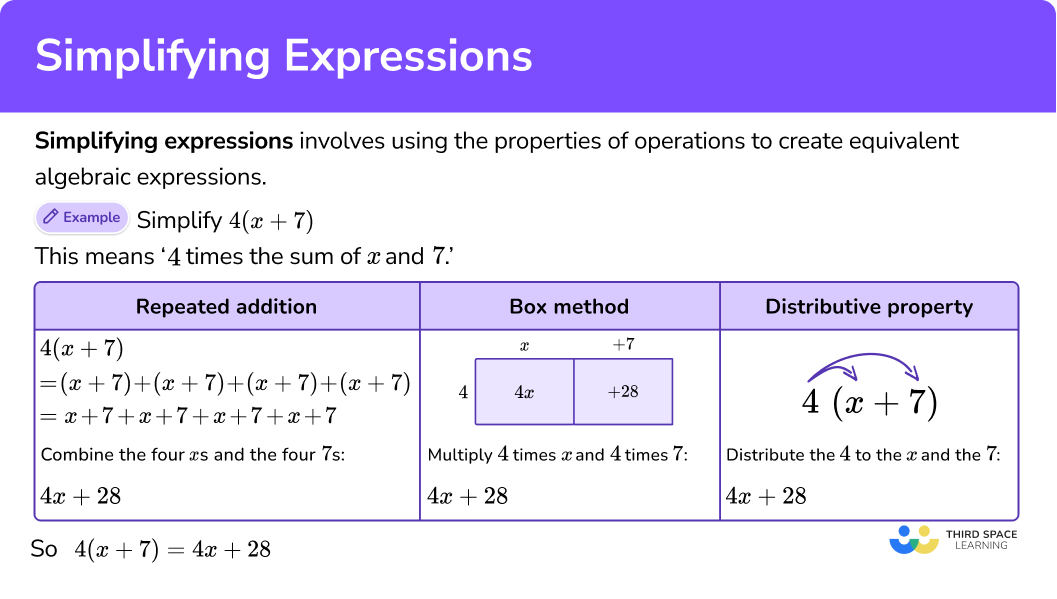## Common Core State Standards

How does this relate to 6th grade math?

• Grade 6 – Expressions and Equations (6.EE.A.3)
Apply the properties of operations to generate equivalent expressions.
For example, apply the distributive property to the expression 3 \, (2 + x) to produce the equivalent expression 6 + 3x; apply the distributive property to the expression 24x + 18y to produce the equivalent expression \, 6 (4x + 3y); apply properties of operations to y + y + y to produce the equivalent expression 3y.

## How to simplify expressions

In order to simplify expressions:

1. Multiply the terms within the parentheses by the term on the outside.
2. Combine like terms.
3. Write the simplified expression.

## Simplifying expressions examples

### Example 1: simplify in one-step

Simplify 3 \, (5 + p).

1. Multiply the terms within the parentheses by the term on the outside.

3 \, (5 + p) is ‘3 times the sum of 5 and p.’

There are 3 different ways to solve:

Notice that each method shows 3 \, (5 + p) = 15 + 3p.

2Combine like terms.

You cannot simplify any further because ‘+3p’ and ‘15’’ are not like terms. One has the variable p, and the other is a constant.

3Write the simplified expression.

3 \, (5 + p) simplified is 15 + 3p.

### Example 2: simplify in two-steps

Simplify 2 \, (2m + 6) + 1.

Multiply the terms within the parentheses by the term on the outside.

Combine like terms.

Write the simplified expression.

### Example 3: simplify in one-step

Simplify 4 \, (8-k) + 3k.

Multiply the terms within the parentheses by the term on the outside.

Combine like terms.

Write the simplified expression.

### Example 4: simplify two-steps with the distributive property

Simplify 9 \, (1 + 2y) - 5y.

Multiply the terms within the parentheses by the term on the outside.

Combine like terms.

Write the simplified expression.

### Example 5: simplify two-steps with two variables with the distributive property

Simplify 7 \, (h + 4t)-9t.

Multiply the terms within the parentheses by the term on the outside.

Combine like terms.

Write the simplified expression.

### Example 6: perimeter multi-step with distributive property

Write the simplified expression for the perimeter of the shape.

Multiply the terms within the parentheses by the term on the outside.

Combine like terms.

Write the simplified expression.

### Teaching tips for simplifying expressions

• Before students can simplify an algebraic expression, they need to understand exactly what makes up the expression. Encourage students to model expressions that have variables with hands-on manipulatives (such as algebra tiles), digital resources or their own drawings before beginning to simplify.

• Even if students have not learned how to operate with integers, they can still be successful at simplifying expressions like these. Encourage students to use what they know about positive and negative numbers and support their understanding with models, like those shown on this page.

### Easy mistakes to make

• Confusing the order of operations (pemdas) when simplifying algebraic expressions
Expressions should always be simplified following the order of operations. For most simple expressions, that means simplifying the parentheses using the distributive property and then adding the like terms from left to right. Doing this out of order, leads to the incorrect answer.

For example,
Simplify 7 \, (x +1)-4x +2x…

• Thinking the original expression and simplified are not equivalent
Just like simplified fractions are equivalent versions of the original fractions, the same is true for simplified algebraic expressions. Using the distributive property and combining like terms does not change the value of the expression.

• Not including a positive or negative sign
When combining the like terms, you must include the sign in front of the term.
For example,
5h + 8p-3h simplified is 2h + 8p.
To combine the like h terms, solve 5h-3h. The subtraction or negative sign stays with the 3h. And the addition or positive sign stays with the 8h.

• Terms with a coefficient of \bf{1}
For terms with a coefficient of 1, you don’t need to write the 1.
For example,

1x=x \hspace{0.6cm} 1y+ 4m=y + 4m \hspace{0.6cm} 6 \, (4 + 1r) = 6 \, (4 + r)

• Forgetting to use parentheses
When multiplying an expression by a value, use parentheses so that each term is multiplied. Not using parentheses changes the outcome.
For example,

2 \, (y+4) = 2y + 8 \hspace{1.3cm} 2 \times y+4=2 y+4

### Practice simplifying expressions questions

1. Simplify 4 \, (d + 10).

4d + 104d + 4044d4 + d + 104 \, (d + 10) is ‘4 times the sum of d and 10.’

There are 3 different ways to solve: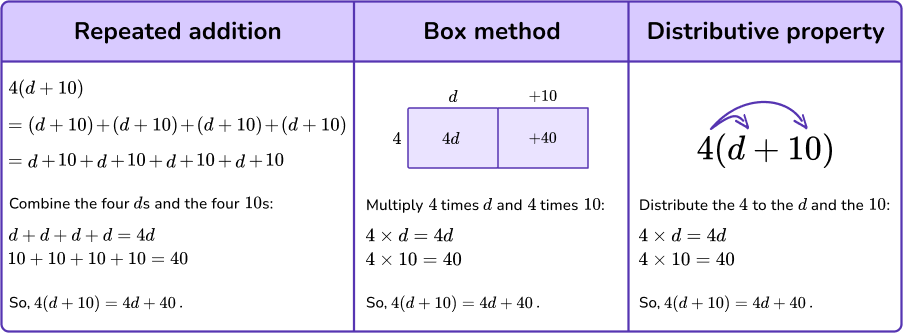2. Simplify 3 \, (1 + 3f)-4f.

5f + 38f5f + 13-fSimplify the parentheses first.

3 \, (1 + 3f) is ‘3 times the sum of 1 and 3f.’

There are 3 different ways to solve: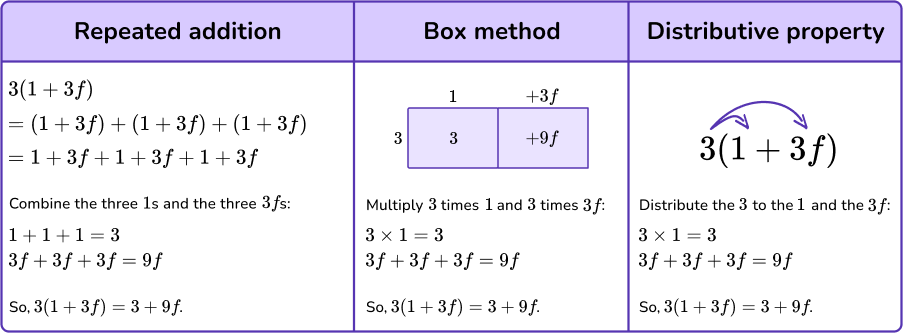You can simplify further because ‘+9f’ and ‘-4f’ are like terms: 9f-4f.

Visually if you have 9 positive f s and then subtract 4 \, f s, there are 5 positive f s left: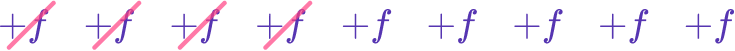So, 9f-4f = 5f.

3 \, (1 + 3f)-4f simplified is 5f + 3.

*Remember, because of the commutative property 3 + 5f = 5f + 3.

3. Simplify 2 \, (x-4) + 9x.

3x11x-43x-411x-8Simplify the parentheses first.

2 \, (x-4) is ‘2 times the difference of x and 4.’

There are 3 different ways to solve: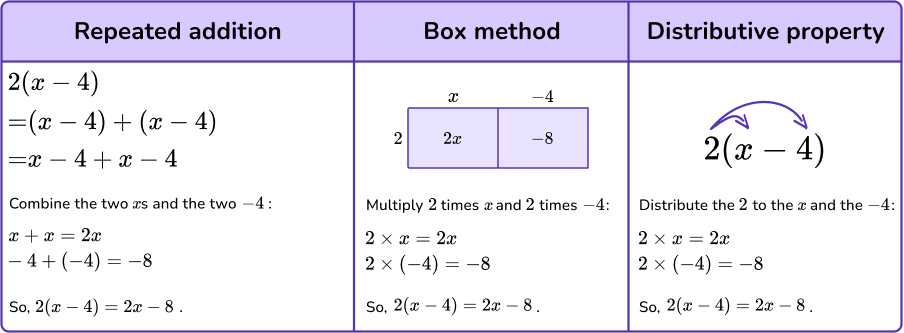You can simplify further because ‘2x’ and ‘+9x’ are like terms: 2x + 9x.

Visually if you have 2 positive x s and then add 9 \, x s, there are 11 positive x s left: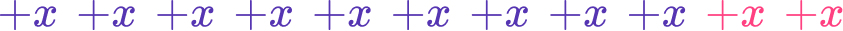So, 2x + 9x = 11x.

2 \, (x-4) + 9x simplified is 11x-8.

4. Simplify 6 \, (4m + 7)-30.

36m24m + 2324m + 1266m-30Simplify the parentheses first.

6 \, (4m + 7) is ‘6 times the sum of 4m and 7.’

Distribute the 6 to the 4m and the 7.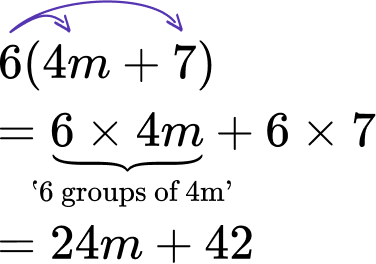So, 6 \, (4m + 7)-30 = 24m + 42-30.

You can simplify further because ‘+42’ and ‘-30’ are like terms: 42-30 = 12.

6 \, (4m + 7)-30 simplified is 24m + 12.

5. Simplify 11 \, (n + 2b)-15b.

11n + 7b18nb11n-13b11b + 7nSimplify the parentheses first.

11 \, (n + 2b) is ‘11 times the sum of n and 2b.’

Distribute the 11 to the n and the 2b.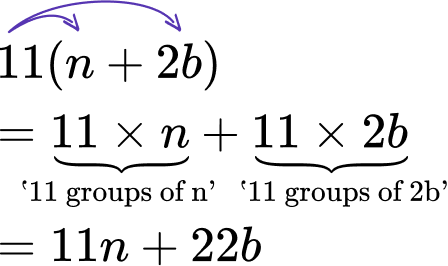So, 11 \, (n + 2b)-15b = 11n + 22b-15b

You can simplify further because ‘+22b’ and ‘-15b’ are like terms: 22b-15b = 7b.

11 \, (n + 2b)-15b simplified is 11n + 7b.

6. Write the simplified expression for the perimeter of the shape.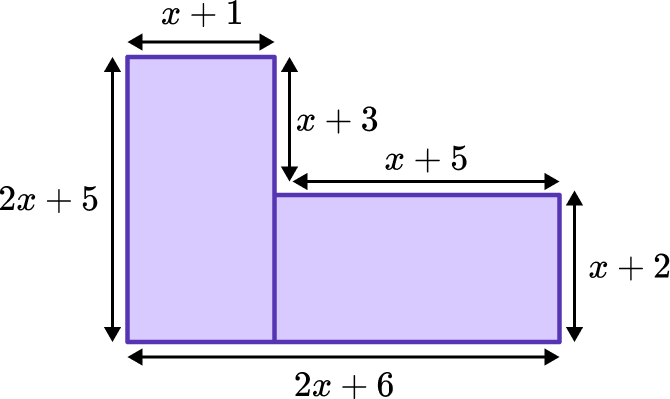8x+226x+144x+114x^{2}+22x+30To calculate the perimeter, add all side lengths together by combining the like terms.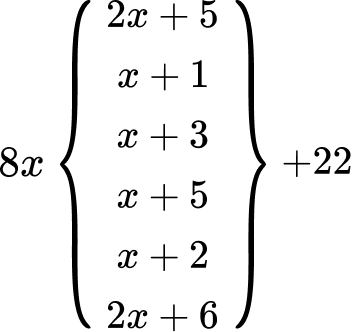The simplified expression for the perimeter is 8x + 22.

## Simplifying expressions FAQs

Is there a way to undo the simplification of an expression?

Yes, you can use factoring to reverse the distributive property.

Can other types of algebraic expressions be simplified?

Yes, in 7th grade, students will learn to simplify rational expressions that are equal to linear equations, including fractions with variables in the numerator and/or the denominator and expressions.

In 8th grade, students will expand on this knowledge by including variables with exponents and continuing into high school, where algebraic expressions are simplified within inequalities.

Can the distributive property be used only with multiplication?

No, the distributive property can be used with multiplication and division. For example,

55 \div 5
=(45+10) \div 5
=45 \div 5+10 \div 5
=9+2
=11

## Still stuck?

At Third Space Learning, we specialize in helping teachers and school leaders to provide personalized math support for more of their students through high-quality, online one-on-one math tutoring delivered by subject experts.

Each week, our tutors support thousands of students who are at risk of not meeting their grade-level expectations, and help accelerate their progress and boost their confidence.